# 三坐标测量软件rational-dmis如何绘制Xbar-R图

SPC:统计分析模块应用统计分析技术对产品进行质量监控和评估计算Ca、Cp、Cpk、Pp、Ppk等重要的生产控制参数可保存为PDF和Exce|两种格式的数据。输出显示：XBar-R图表、XBar-S图表、极限图表、运行时间图表、柱状图表，并输出统计数据。只需一个鼠标拖执操作，即可完成要统计的元素 SPC。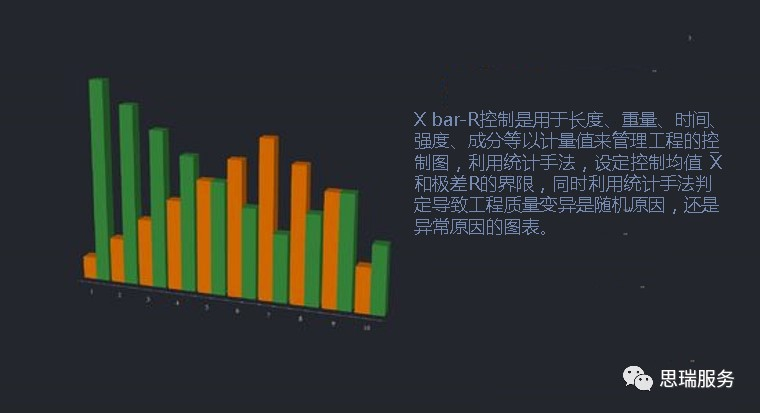实验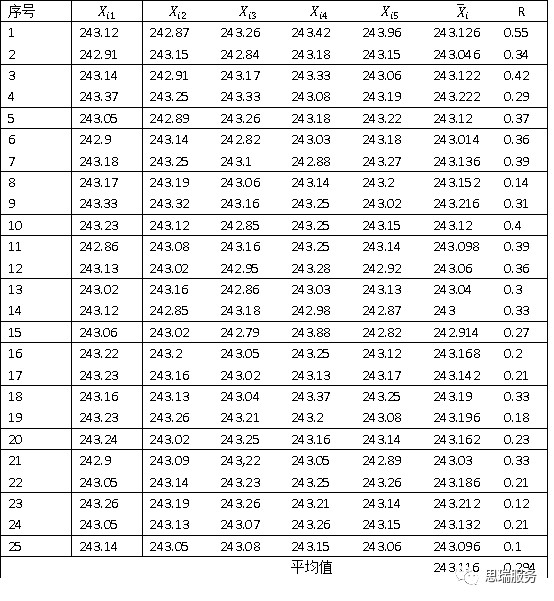Xbar=(X1+X2+X3+...Xn)/N

R=Xmax-Xmin

Xbar图：CL==243.116

UCL=+R=243.116+0.58*0.294=243.29   X均值上限

LCL=-R=243.116-0.58*0.294=242.95    X均值下限

R图：CL=R=0.294

UCL=R=2.11*0.294=0.622      极差上限

LCL =<0                                 极差下限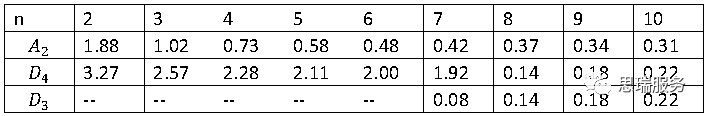*对于样本容量小于7的情况,可能技术上为一个负值，即没有下限。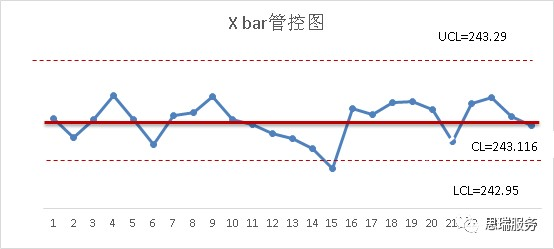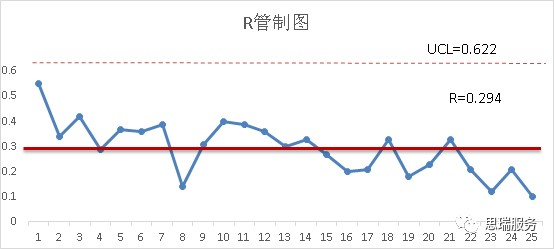•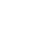返回顶部